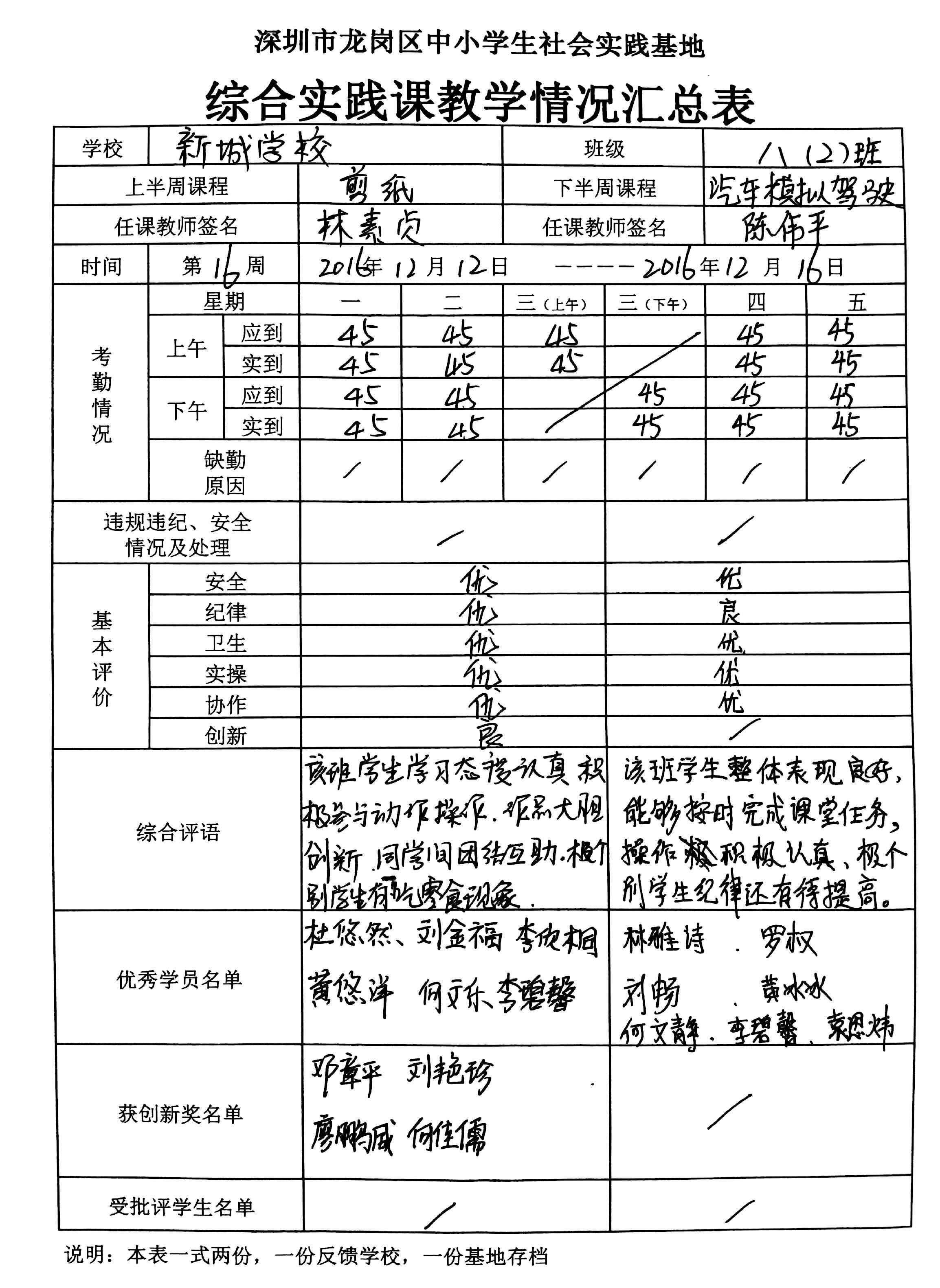# 802班综合实践课精彩瞬间及考核反馈（12月社会实践）

## 综合实践课篇30-考核反馈

 综合实践课行为表现等级（第16周考核） 编号 姓名最后一个字 剪纸等级 驾驶等级 综合表现考核等级 1 宇 A+ A+ A 2 炯 A+ A- A- 3 平 A+ A+ A 4 然 A+ A+ A 5 儒 A+ A+ A 6 静 A+ A+ A 7 乐 A+ A+ A 8 峰 A A+ A- 9 薇 A+ A+ A 10 冰 A+ A- A 11 洋 A+ A+ A 12 豪 A- A+ A- 13 昊 A+ A+ A 14 馨 A+ A+ A 15 颖 A+ A+ A 16 雅 A+ A+ A 17 桐 A+ A- A 18 欢 A+ A+ A 19 威 A+ A+ A 20 钰 A+ A+ A 21 诗 A+ B+ A 22 俊 A+ A+ A 23 东 A+ A+ A 24 福 A+ A+ A 25 珍 A+ A- A 26 权 A+ A+ A 27 城 A+ A+ A- 28 晶 A+ B+ A 29 东 A+ A+ A 30 林 A+ A+ A 31 乐 A+ A+ A 32 煌 A+ A+ A 33 琳 A+ A+ A 34 怡 A+ A+ A 35 慧 A+ A- A 36 威 A+ A+ A 37 炜 A+ A- A- 38 桐 A A+ A 39 颖 A+ A+ A 40 炜 A+ A+ A 41 畅 A+ A+ A 42 基 A+ A A 43 乾 B+ A+ A 44 灼 A+ B- A 45 才 A+ A+ A• 本文由 发表于 2016年12月13日21:32:57
• 转载请务必保留本文链接：https://www.ky125.com/2016/12/13/2842/